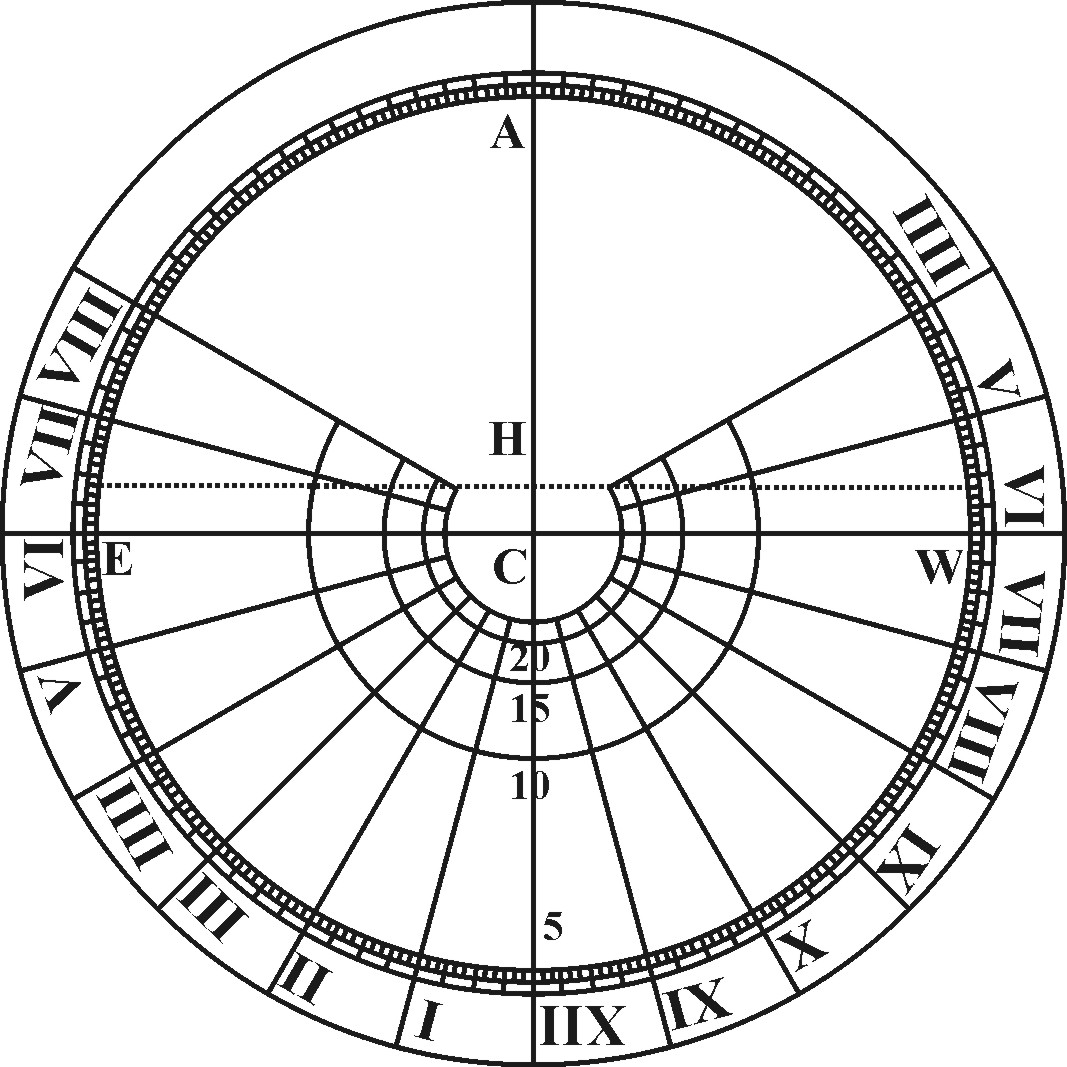CHAP. I.To draw the Hour-lines in an Equinoctial Plane.

An Equinoctial Plane is that which is parallel to the Equinoctial Circle here represented by EAV, wherein the spaces between the Hour-circles being equal, there is no need of further Precept, but only to draw a Circle, and to divide it into 24 equal parts from the 24 Hours, and subdivide each Hour into Halves and Quarters, and then to set up the Style perpendicular to the Plane in the Center of the Circle. The help which these Lines of Proportion do here afford us, is only in the division of the Circle, which may be done readily by that which I shewed before in the First Book of the Sector.

For Example: Suppose the Semidiameter of the Equinoctial Circle to be six Inches, and that it were required to know the distance of the Hour-points each from other; here each Hour being 15 gr. distant from other, I extend the Compasses from the Sine of 50 gr. unto the Sine of 7 gr. 30 m. the half of 15 gr. and I find the same extent to reach in the Line of Numbers from 6.00 unto 1.56.

Or in cross work I extend them from the Sine of 30 gr. unto 6.00 in the Line of Numbers, the same extent will reach from the Sine of 7 gr. 30 m. unto 1.56 in the Line of Numbers; which shews that in a Circle of six Inches semidiameter, the distance of the Hour-points each from other will be about 1 Inch 56 Centesms or parts of 100. The like reason holds for the inscribing of all other Chords in the Prop. following.Zurück zum Inhaltsverzeichnis Nächstes Kapitel© Rainer Stumpe URL: http://www.rainerstumpe.de